• Call Now

1800-102-2727•

# Kaleidoscope

Have you seen yourself in the two perpendicular mirrors? You can get these perpendicular mirrors at barber shops when you go for a haircut. Normally when you see yourself in a plane mirror a single image is formed behind the mirror. Now when you see yourself in a two mirrors system where the mirrors are placed at some angle then there will be more than one image. The reason behind it is that when two plane mirrors are at some angle then the image formed by one mirror behaves as an object for the second mirror. Thus the images formed are because of multiple reflections. Using a similar concept an optical playing toy is formed named a kaleidoscope. Let's see what a kaleidoscope is, in detail !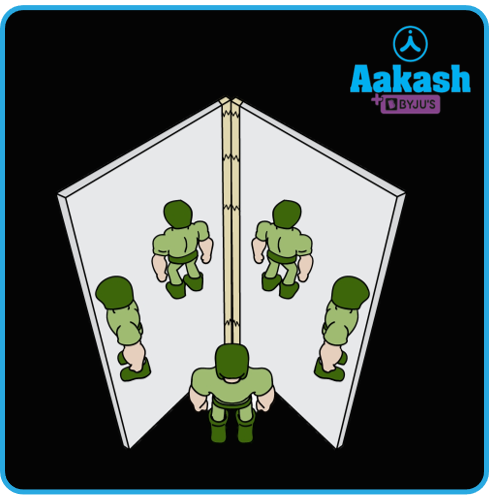Table of content

• Kaleidoscope
• Working Principle of kaleidoscope
• Making of Kaleidoscope
• Practice problem
• FAQs

## Kaleidoscope

Kaleidoscopes are entertaining toys like optical devices. It consists of two or more reflecting surfaces that are tilted at some specified angle to one another. When viewed from the other end of the kaleidoscope, this helps to produce symmetrical patterns. Many symmetrical patterns can be created by rotating the tube, which is covered in reflecting surfaces made of coloured glass fragments.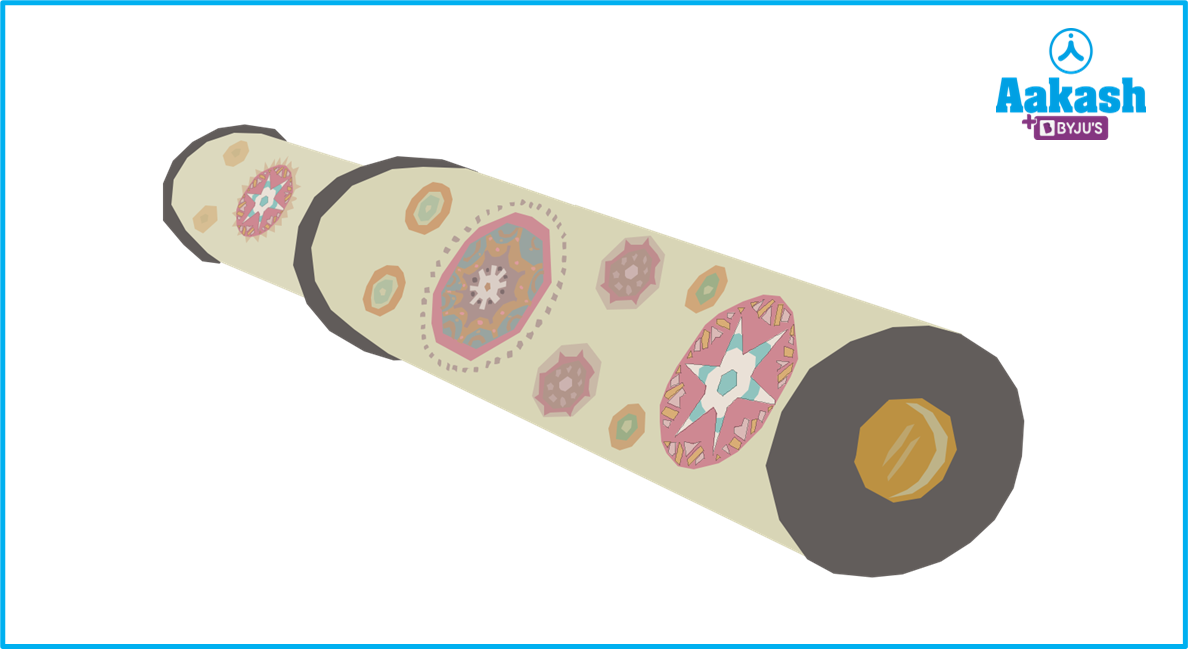In 1817, Scottish inventor David Brewster created the kaleidoscope. It is made up of the words "Kalos" and "eidos," which respectively mean "what is seen" and "beautiful". Here, "skopeo" simply means "to glance".

The glass and coloured beads inside the kaleidoscope reflect sunlight. Within the tube, the light refracting off the mirrors creates lovely, captivating patterns. The viewer gazing into the kaleidoscope finds these lovely patterns to be a visual treat. In truth, a kaleidoscope produces a variety of beautiful images as the tube is rotated because patterns vary and blend into one another. A snip of the pattern by kaleidoscope is shown in the figure below.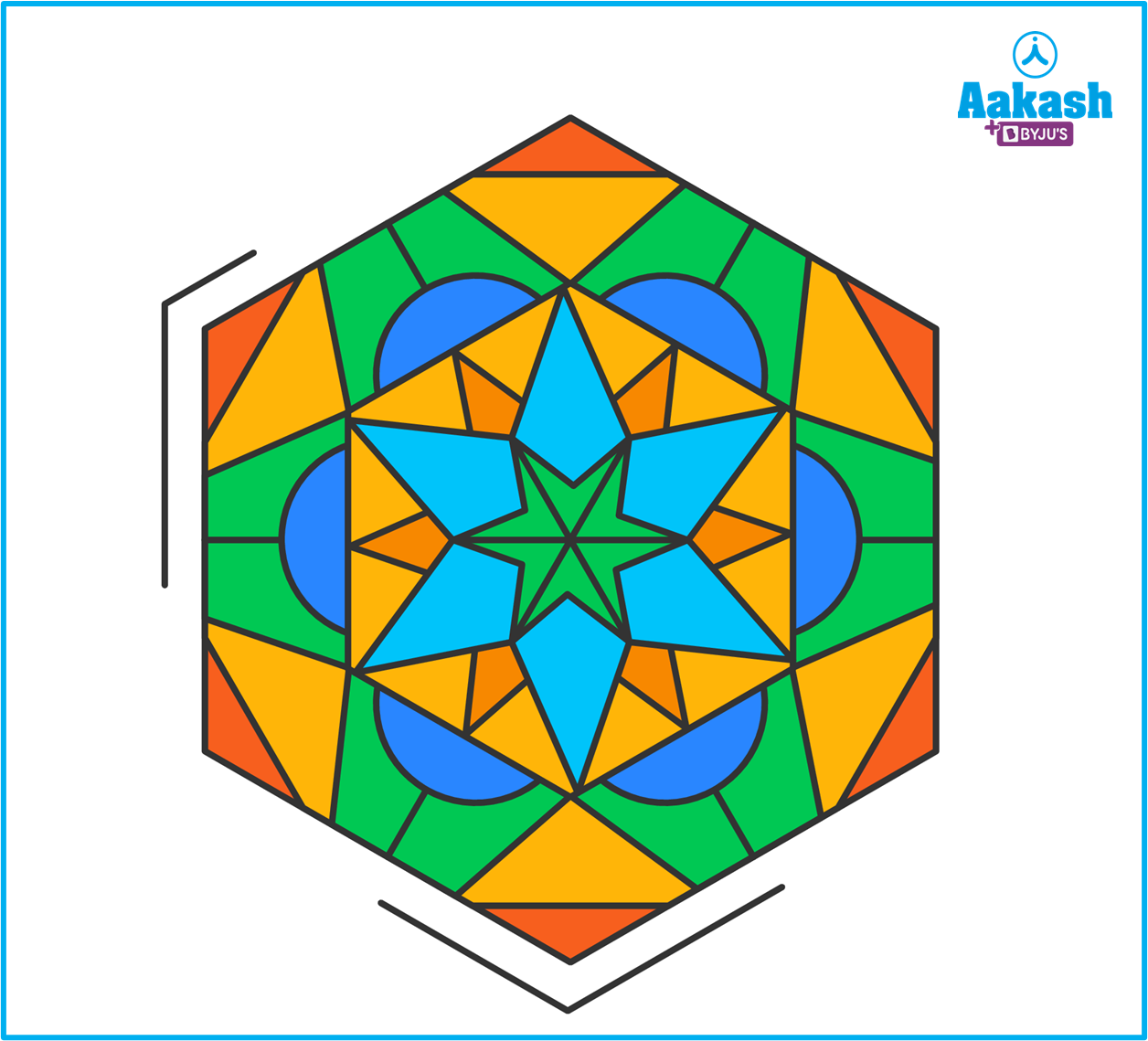## Working Principle of kaleidoscope

The basic principle of kaleidoscope is the laws of reflection of light,

1. The angle of incidence and the angle of reflection are the same.
2. All three: the incident ray, the reflected ray, and the normal to the surface are on the same plane.

The angle between the mirrors of the kaleidoscopes should be acute. The number of images formed in a kaleidoscope depends on the angle between the two mirrors.

If an object is put between two plane mirrors M1 and M2, and the mirrors are inclined to each other at an acute angle, we may check two things to determine how many images can be produced by the system. One is $\frac{{360}^{0}}{\theta }$ and whether the position of the object is symmetrical or asymmetrical to the system. We have two cases based on these two standards:

Case 1: If $\frac{{360}^{0}}{\theta }$is even

Number of images formed, n=$\frac{{360}^{0}}{\theta }$-1

This is true for both symmetric and asymmetric positions of objects.

Case 2: If $\frac{{360}^{0}}{\theta }$ is odd

If the result is odd, we must determine whether the object is positioned symmetrically or asymmetrically with respect to the system.

• If the object is placed symmetrically to the system, then the number of images formed by this system, n=$\frac{{360}^{0}}{\theta }$-1
• If the object is placed asymmetrically to the system, then the number of images formed by this system, n=$\frac{{360}^{0}}{\theta }$

## Making of Kaleidoscope

We may make our own kaleidoscope and enjoy seeing the different hues and patterns it produces. The idea of reflection of light forms the foundation of the kaleidoscope. Following the techniques outlined below, we can create a kaleidoscope for free. For making the kaleidoscope we need the following things :

• At least three or more equal-sized mirrors or plane reflective surfaces
• A cylindrical cardboard box, or plastic pipe
• One scissors
• A pencil
• A plate of glass
• a cardboard sheet

Now take three mirrors of the same size. The three mirrors should be glued together along their length so that the reflecting surfaces are facing inward and the painted surfaces are facing outward. These mirrors are angled at a 60-degree angle to one another, creating a hollow prism as shown in the figure.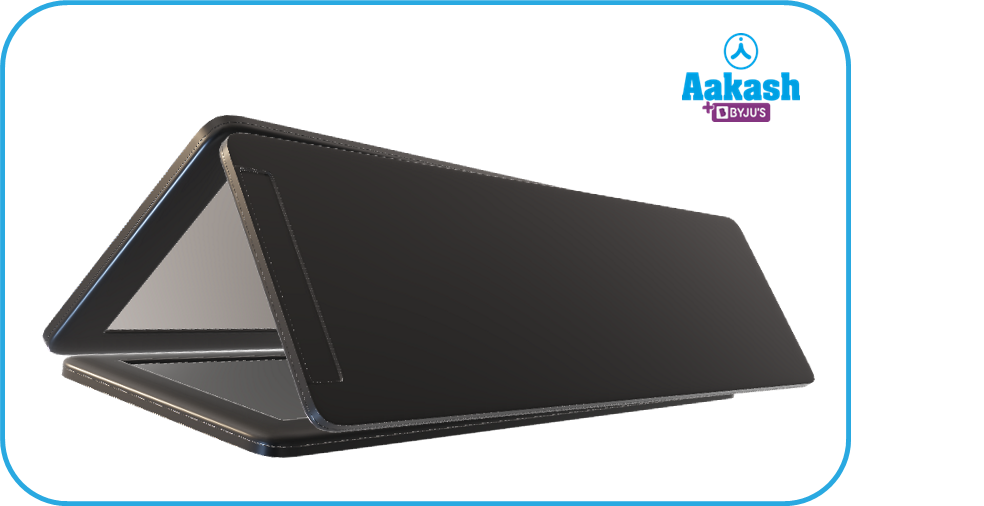The glued combination of the mirrors should be placed inside a cylindrical cardboard box that has a length bigger than the length of the mirrors. Put some pieces of bangles, colourful beads and sprinkles on one side of the cylinder and capped this end with one circular glass plate of the same size as the cylinder so that light can pass through it. Now cut a circular cardboard piece of the same size as the circular portion of the cylinder and put it on the other side of the cylinder with the help of the glue. This circular board can be looked inside by making a hole in it with a pencil. You can use your kaleidoscope now. Enjoy the complex patterns that are created inside the kaleidoscope by rotating the box.

## Practice problem

Q. Two plane mirrors are inclined at an angle of 45 degrees. Find the number of images formed by the mirrors, if the object is placed symmetric to both the mirrors.

A. Given =45o

Now, check $\frac{{360}^{0}}{\theta }=\frac{{360}^{0}}{{45}^{o}}=8$ is even

So the number of images formed, N=$\frac{{360}^{0}}{\theta }$1

N=$\frac{{360}^{0}}{\theta }$45o-1

N=8-1

N=7

Hence the number of images is 7.

Q. If a man stands symmetrically in front of the two mirrors which are placed side by side at an angle of 72 degrees. Find the number of image forms.

A. Given $\theta ={72}^{o}$

Now, check $\frac{{360}^{0}}{\theta }=\frac{{360}^{0}}{{72}^{o}}=5$ is odd.

As the object is placed symmetrically, the number of images formed is

$N=\frac{{360}^{0}}{\theta }-1$

$N=\frac{{360}^{0}}{\theta }-1$

N=5-1

N=4

Hence the number of images formed is 7.

Q. Two plane mirrors are placed at an angle of 40 degrees. If an object is placed asymmetrically in between these two mirrors, what is the number of images in these mirrors?

A. Given $\theta ={40}^{o}$

Now check $\frac{{360}^{0}}{\theta }=\frac{{360}^{0}}{{40}^{o}}=9$ is odd.

As the object is placed asymmetrically so, the number images formed is

$N=\frac{{360}^{0}}{\theta }$

$N=\frac{{360}^{0}}{{40}^{o}}$

N=9

Hence the number of images formed is 9.

Q. Two inclined plane mirrors are forming images of an asymmetrically placed object. If the angle between the mirrors is 36 degrees what is the number of images?

A. Given $\theta ={36}^{o}$

Now check $\frac{{360}^{0}}{\theta }=\frac{{360}^{0}}{{36}^{o}}=10$ is even

So the number of images $N=\frac{{360}^{0}}{\theta }-1$

$N=\frac{{360}^{0}}{{36}^{o}}-1$

N=10-1

N=9

Hence the number of images formed is 9.

## FAQs

Q. What is the angle between the two adjacent plane mirrors in a kaleidoscope if there are 4 mirrors used?
A.
We know that, usually in a kaleidoscope the mirrors are of the same size. When 4 mirrors are used, they will form a square. So the angle between any two adjacent mirrors will be 90 degrees. So, each pair of mirrors will make $\frac{360}{90}-1=$3 images of the objects placed between them.

Q. What is the use of the kaleidoscope?
A.
kaleidoscope is an optical device which is used to create beautiful patterns. These patterns are sometimes used by fashion designers. The kaleidoscope is also used as an entertainment toy.

Q. What is the unique feature of the kaleidoscope?
A.
The interesting fact about the kaleidoscope is that similar patterns are not seen again. So it is used to get the new idea of a pattern for conceptualising new design. Actually the objects and their positions are less likely to be the same when the kaleidoscope is rotated. So each time a new design comes up.

Q. What is the periscope? Is it similar to a kaleidoscope?
A.
Periscope is different from the kaleidoscope. Periscope is an optical device used mainly by the navy to see above the surface of the ocean while inside the submarine. It has two plane mirrors placed at 45 degree to the horizontal as shown in the figure, which helps in seeing the objects floating in the sea.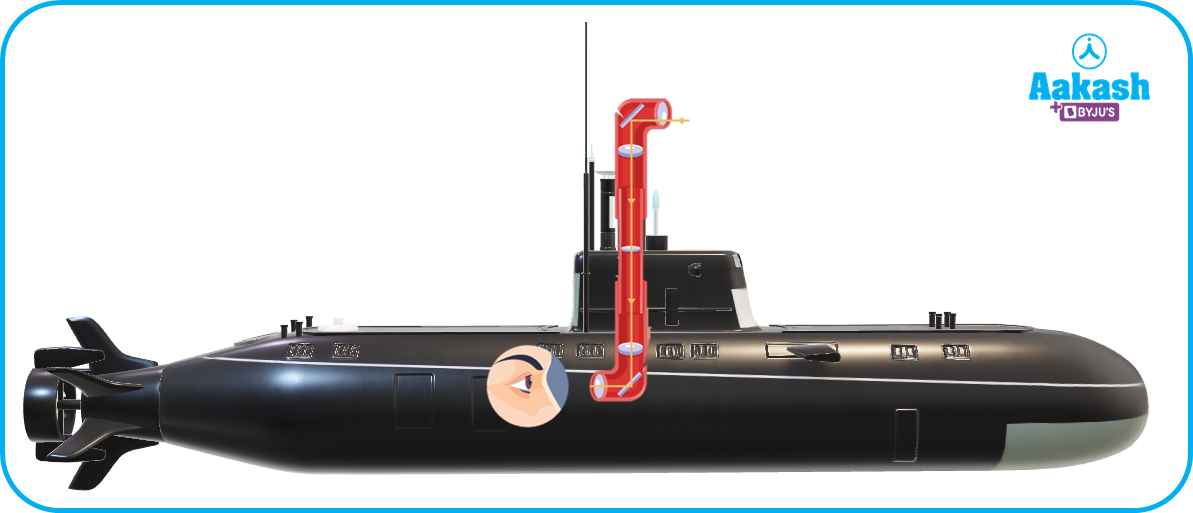Talk to our expert
Resend OTP Timer =
By submitting up, I agree to receive all the Whatsapp communication on my registered number and Aakash terms and conditions and privacy policy